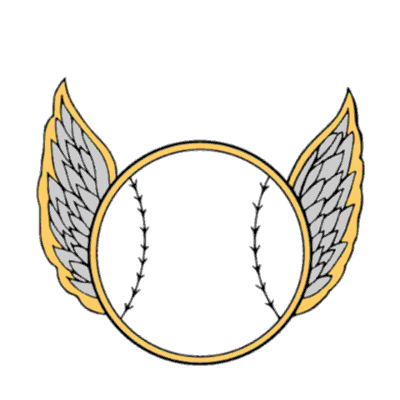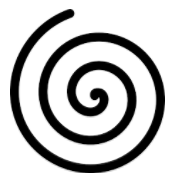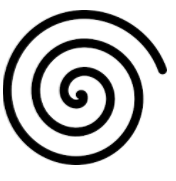# J'ai une couche d'entités polygonale que je voudrais numeroter automatiquement mais en spiral (En escargot). De facon à avoir une continuité par voisinage. je suis un debutant en python, merci de m'aider. Merci

1505
26
02-21-2018 12:42 PMNew Contributor II

J'ai une couche d'entités polygonale que je voudrais numéroter automatiquement mais en spirale (En escargot). De facon à avoir une continuité par voisinage. je suis un débutant en python, merci de m'aider. Merci

Tags (2)
26 RepliesbyMVP Regular Contributor

Translation: I have a layer of polygonal entities that I would like to automatically number but spiral. In order to have continuity by neighborhood. I am a beginner in python, thank you for helping me. Thank you

Question: Do you want the spiral in a clockwise or counterclockwise direction? ( Voulez-vous la spirale dans le sens des aiguilles d'une montre ou dans le sens contraire?)New Contributor II

Thank you, I would like to have a spiral in a clockwise !

Many thanks!byMVP Regular Contributor

See Dan Patterson‌'s comment in this thread: numbering polygon in clockwise.  Although the message doesn't have a code example, it gives a suggestion on how this problem might be approached.New Contributor II

Yes, I'm trying to understand his approach to adapting it. But it will not be for tomorrow.

ThanksbyMVP Esteemed Contributor
``````def angle_2pnts(p0, p1):
"""Two point angle
: angle = atan2(vector2.y, vector2.x) - atan2(vector1.y, vector1.x);
"""
ba = p1 - p0
ang_ab = np.arctan2(*ba[::-1])
return np.rad2deg(ang_ab % (2 * np.pi))

p1 = np.array([1, 1])

p0 = np.array([0, 0])

angle_2pnts(p0, p1)
45.0‍‍‍‍‍‍‍‍‍‍‍‍‍‍‍``````

Sadly for some, I use numpy to perform all my calculations, but this may help

p0... consider that your center point

p1.... pN  are all your other points.

If you had a list of points, I could modify my example or you could translate this into straight python using elementary geometry

In short, the angles are relative to the X-axis ranging from 180 to -180... sort in descending order to give you a clockwise direction, sort in ascending order to get counterclockwise.

If you have data in a particular form, I can provide a field calculator expression, and I have a toolbox somewhere that does radial, lexicographic and other sorting types if needed (I have probably done a blog post at some time as well)New Contributor II

Thank you Dan,

what do you think if I send you an example of a shapefile.

I can't apply it to my polygons.

Many thanksbyMVP Esteemed Contributor

Ok... I found some field calculations.

If you have a featureclass/shapefile, this field calculator will do the calculations for you.

You must have projected coordinates!

If you copy that file into a text editor and save it with as .... azimuth_to.cal .... you can load it up into the field calculator.  It is the same principle, but it does the azimuth to... but you can then sort it using

Sort .... since you are only sorting on a regular field.

Normally the Sort tool (in Map or PRO) is only available at the advanced license if you want to sort on the Shape field or to use multiple fields.

``````# -*- coding: UTF-8 -*-
"""-----------------------------------------
Input shape field: returns angle between 0 and <360 based upon the first and last point
azimuth_to(!Shape!,from_x, from_y, from_north=True)
ie azimuth_to(!Shape!, 300050, 5000050, True)
"""
import math
def azimuth_to(shape, from_x, from_y, from_north):
x = shape.centroid.X
y = shape.centroid.Y
radian = math.atan2((y - from_y), (x - from_x))
if from_north:
angle = (450 - angle) % 360
return angle
__esri_field_calculator_splitter__
azimuth_to(!Shape!, 300050, 5000050, True)‍‍‍‍‍‍‍‍‍‍‍‍‍‍‍‍‍‍‍‍‍‍‍‍‍‍‍‍‍‍‍‍‍‍‍‍‍‍‍‍‍‍‍‍‍‍‍‍‍‍‍``````

NOW  see the last line... the 300050 and 5000050 are the centre X and Y values... you will need to use the python parser in the field calculator and determine and enter your central points.  If you want them from North, then entre True, otherwise you get the angles from the X-axis.

If set to False, then you have to sort in descending order.

if set to True, then you will be sorting in ascending order.

Hope this is clear as mud... draw a picture if it helps.byEsri Esteemed Contributor

Hi Ned Charles ,

If you want to number them as a "spiral", I assume that distance from the first (center) also influences.the resulting numbering as well as the start angle:or for instance this wayCan you provide a screenshot or sample of your data to see what it looks like?New Contributor II
20/5000
image in attachment

The parcels are in red and the black line is the neighborhood numbering direction.